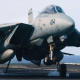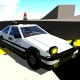## AUTO-AIMED TURRET AK-2100

12.2k SenSkysh  7 months ago
23,948 downloads
Auto Credit Based on SenSkysh's ak-2100### A little about the formula

#### Horizontal rotator:

`(TargetHeading - Heading + rate(TargetHeading)*(((1000 * sin(asin((TargetDistance * 9.81)/(pow(1000, 2)))/2))/9.81) * 2))/180`
`1000` here is the projectile speed of the cannon

#### Vertical rotator:

`clamp((TargetElevation + rate(TargetElevation)*(((1000 * sin(asin((TargetDistance * 9.81)/(pow(1000, 2)))/2))/9.81) * 2)) + (asin(((TargetDistance + rate(TargetDistance)*(((1000 * sin(asin((TargetDistance * 9.81)/(pow(1000, 2)))/2))/9.81) * 2))* 9.81)/(pow(1000, 2)))/2),-10,45)/45`
`1000` here is the projectile speed of the cannon, `-10,45` - limiting angles of guidance

#### Upd.

Time does not stand still and gradually I came to a more sophisticated formula. She is somewhat more accurate

##### Horizontal:

```(TargetHeading - Heading + (rate(TargetHeading)) * (((v * sin(asin((TargetDistance * 9.81)/(pow(v, 2)))/2))/9.81) * 2) )/angle```
where
`v` - projectile speed
`angle` - rotator range of motion

##### Vertical:

`clamp(TargetElevation + (rate(TargetElevation)) * (TargetDistance + rate(TargetDistance)*TargetDistance/v)/v + (asin((cos(TargetElevation + (rate(TargetElevation)) * (TargetDistance + rate(TargetDistance)*TargetDistance/v)/v) * (TargetDistance + rate(TargetDistance)*TargetDistance/v) * 9.81)/(pow(v, 2)))/2) -PitchAngle*cos(TargetHeading - Heading + (rate(TargetHeading)) * (TargetDistance + rate(TargetDistance)*TargetDistance/v)/v) - RollAngle*sin(TargetHeading - Heading + (rate(TargetHeading)) * (TargetDistance + rate(TargetDistance)*TargetDistance/v)/v),nA,pA)/angle`
where
`v` - projectile speed
`nA` - negative vertical guidance
`pA` - positive vertical guidance
`angle` - rotator range of motion

#### Upd2

Just for accuracy#### General Characteristics

• Predecessor ak-2100
• Successors 7 airplane(s) +77 bonus
• This plane has been featured
• Created On Android
• Wingspan 16.4ft (5.0m)
• Length 26.8ft (8.2m)
• Height 16.1ft (4.9m)
• Empty Weight 149,236lbs (67,692kg)
• Loaded Weight 155,289lbs (70,438kg)

#### Performance

• Wing Loading 439,839.3lbs/ft2 (2,147,483.6kg/m2)
• Wing Area 0.0ft2 (0.0m2)
• Drag Points 8812

#### Parts

• Number of Parts 88
• Control Surfaces 0
•12.2k SenSkysh

I already wrote that the free-use formula

Pinned 2 months ago
•Yes

23 days ago
•@SenSkysh cool

23 days ago
•12.2k SenSkysh

@MrShenanigans yeah

23 days ago
•@SenSkysh oh uh is it also possible if I use this on a like a ship or a destroyer?

23 days ago
•12.2k SenSkysh

@Joeyjag missiles do not stand out as a target, therefore it is impossible

26 days ago
•135 Joeyjag

Is there any way to make an auto Turret that locks onto missiles/other projectiles just like a cwis? I've been trying to figure out if it was possible but am not very experienced with code

+1 27 days ago
•2,885 frank77831

May I use your Rotator on my work? I want to make a WWII Bomber plane with auto-attack guns

one month ago
•5,514 TrueNoMan

@SenSkysh Kay then

one month ago
•12.2k SenSkysh

@TrueNoMan `angle` - 90. `min` I advise you to change to 1

one month ago
•5,514 TrueNoMan

@SenSkysh Another question. For example, my vertical rotator has a range of 90˚, but the `min` setting in XML had been changed to 0 which means I can only turn it upward by 90˚ but I can't turn it downward. In this case, what should I fill into `angle`?

one month ago
•12.2k SenSkysh

@TrueNoMan yes, for example the t-55 can lower the gun by 5 degrees and raise it by 18
nA = -5, pA = 18

one month ago
•5,514 TrueNoMan

@SenSkysh So, um, should I fill in the max angle of my rotator turning upwards in pA and the max angle of my rotator turning downwards in nA?

one month ago
•12.2k SenSkysh

@TrueNoMan I didn't quite understand what you meant. min and max - limiting rotation in degrees so that the cannon does not pass through the roof, for example. Range - how many degrees the rotator will turn if in input 1

one month ago
•5,514 TrueNoMan

@SenSkysh the min, max and range in Overload you mean?

one month ago
•12.2k SenSkysh

@TrueNoMan elevation

one month ago
•5,514 TrueNoMan

@SenSkysh What's the meaning of "vertical guidance"? Elevation and depression angle?

one month ago
•20.7k SyntheticL

@SenSkysh Hello, could I use the formula in this gun for my 76mm Naval gun on a project? You will get credit of course!

2 months ago
•@SenSkysh ok

2 months ago
•12.2k SenSkysh

@MrShenanigans It's my pleasure

2 months ago
•@SenSkysh since youhelped me with the code i would like too give you credit cause the b29 has auto aiming computer turrets

2 months ago
•12.2k SenSkysh

@MrShenanigans I did not quite understand

2 months ago
•@SenSkysh can i give you credit on my b29 cause the turrets have auto aiming on them

2 months ago
•Can you make a version of this that its in a vehicle? I tried to make it but it messes up the aim

2 months ago
•@SenSkysh oh ok

3 months ago